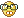We are here with you hands in hands to facilitate your learning & don't appreciate the idea of copying or replicating solutions. Read More>>

#www.vustudents.ning.com

 www.bit.ly/vucodes + Link For Assignments, GDBs & Online Quizzes Solution www.bit.ly/papersvu + Link For Past Papers, Solved MCQs, Short Notes & More

Dear Students! Share your Assignments / GDBs / Quizzes files as you receive in your LMS, So it can be discussed/solved timely. Add Discussion

Assignment # 3 Discussion Spring 2012

Question No. 1 :        [ 5 * 2 = 10 marks]

Suppose following is a work sheet of MS Excel.

Apply the appropriate formulas in MS Excel using the same data given in above diagram, to find the result (same as shown in the diagram) of the below mentioned requirements and just write down the formulas in the solution file ( MS word file).

1. Sum of Marks of all subjects
2. Average of all Marks
3. Highest Marks attained
4. Total number of subjects having Grade A
5. Sum of Marks of those subjects having Grade B

Question No. 2 :        [ 5 marks]

Write JavaScript code using “For loop” to display all the odd numbers between 1 and 100 (both inclusive). Each number should be displayed on a separate line as follows:

1

3

5

7

9

11

13

.

.

.

.

.

93

95

97

99

Solution 1:

Find Result of                                  Formulas in MS Excel

Sum of marks of all subjects          =SUM(B6:B15)

Average of all marks                      =AVERAGE(B6:B15)

Highest marks attained                   =MAX(B6:B15)

Total number of subjects having Grade A             =COUNTIF(C6:C15,"A")

Sum of Marks of those subjects having Grade B   =SUMIF(C6:C15,"B",B6:B15)

Solution 2:

<html>

<title>For loop</title>

<body>

<script>

for(i=1;i<=99;i=i+2) {

document.write(i+"<br>");

}

</script>

</body>

</html>

+ How to Join Subject Study Groups & Get Helping Material?

+ How to become Top Reputation, Angels, Intellectual, Featured Members & Moderators?

+ VU Students Reserves The Right to Delete Your Profile, If?

Views: 2035

.

+ http://bit.ly/vucodes (Link for Assignments, GDBs & Online Quizzes Solution)

+ http://bit.ly/papersvu (Link for Past Papers, Solved MCQs, Short Notes & More)

### Replies to This Discussion

your style of formula for those who are good in excel are already have some how know which is not easy to understand for beginner.

I think so for the rest I don't know their views.

@Afzal

Answer depends on which Rows and Columns Numbers you are using. According to assignment picture, your solution is wrongAssignment Solved, Solution UploadedNote: below is according to my excel format so, please see you cell reference and do accordingly.

=SUM()
=SUM(B6:B15)

=AVERAGE()
=AVERAGE(B6:B15)

=MAX()
=MAX(B6:B15)

=COUNTIF()
=COUNTIF(C6:C15,”A”)

=SUMIF()
=SUMIF(C6:C15,C7,B6:B15)

thanks.